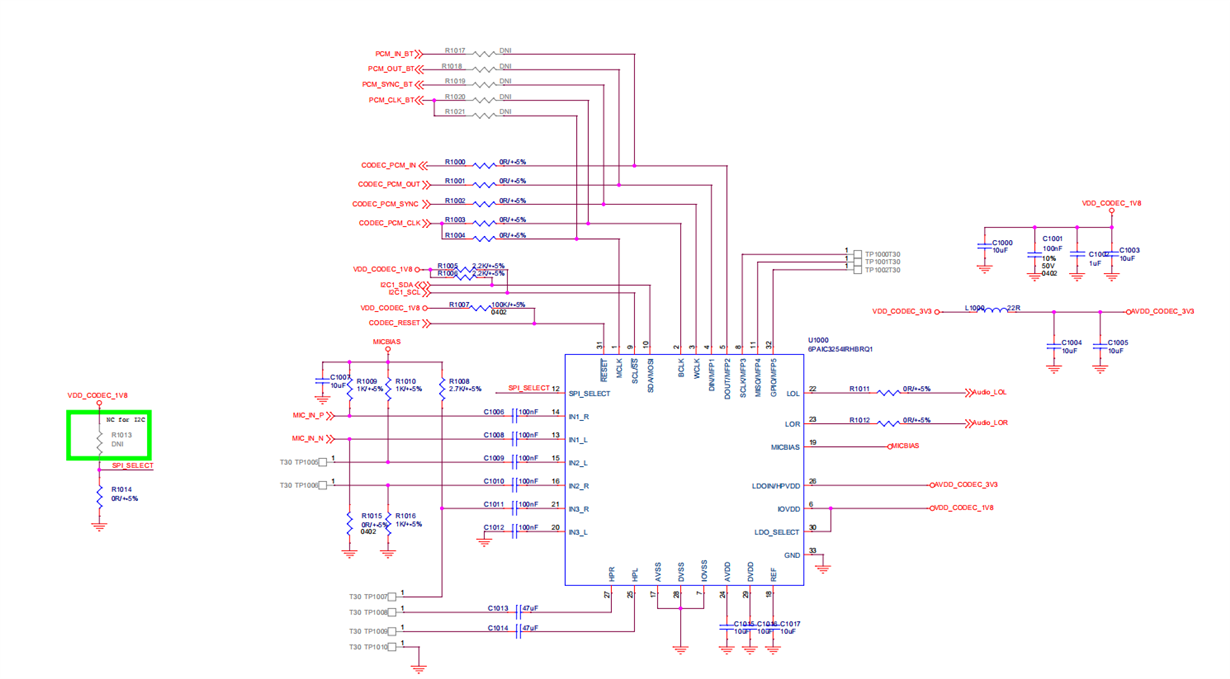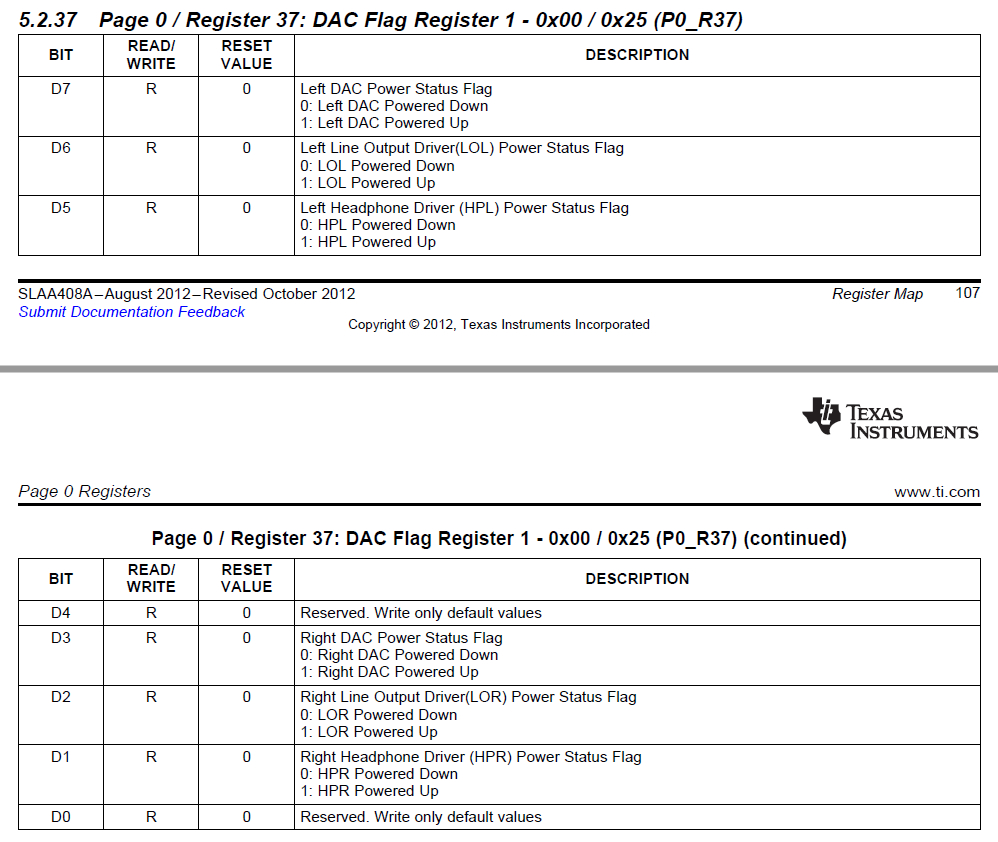If you have a related question, please click the "Ask a related question" button in the top right corner. The newly created question will be automatically linked to this question.

# TLV320AIC3254-Q1: TLV320AIC3254-Q1

Part Number: TLV320AIC3254-Q1

Hi ，

I had used  codeic IC -TLV320AIC3254-Q1 to debug the audio driver on linux platform , there is a problem that when I play audio , the Audio_LOL and Audio_LOR has no anything out .

I had tested power of codec IC and is ok,.when play audio file ,the waveform of DINMFP1 \ BCLK \ LOL\LOR  as below:the value of register as below:add is register address , value is the value of register

mike < write i2c add=0x0 value=0x0, iRet=0 >
mike < write i2c add=0xd value=0x0, iRet=0 >
mike < write i2c add=0xe value=0x80, iRet=0 >
mike < write i2c add=0x13 value=0x83, iRet=0 >
mike < write i2c add=0x1e value=0x18, iRet=0 >
mike < write i2c add=0x0 value=0x1, iRet=0 >
mike < write i2c add=0xe value=0x8, iRet=0 >
mike < write i2c add=0xf value=0x8, iRet=0 >
mike < write i2c add=0x0 value=0x0, iRet=0 >
mike < write i2c add=0x3f value=0x98, iRet=0 >
mike < write i2c add=0x3f value=0xd8, iRet=0 >
mike < write i2c add=0x0 value=0x1, iRet=0 >
mike < write i2c add=0x9 value=0x8, iRet=0 >
mike < write i2c add=0x9 value=0xc, iRet=0 >
mike < write i2c add=0x12 value=0x0, iRet=0 >
mike < write i2c add=0x13 value=0x0, iRet=0 >

and the schematic of audio as below:so why when play audio , the LOL/LOR has no anything out ?

there are some registers to be set up?

• Hello User,

I would recommend that we first use the internal beep generator function to establish that the clocks and hardware connections are proper. The procedure is documented in this application report. The procedure is pretty specific and please follow as directed.

If you are still having problems, provide a dump of all the register configurations in page 0 and page 1.

Best Regards.

• Hello ，

I have tested the Beep Generator of  the appplication repoort but the LOL and LOR has no any signal output ,registers of  the Beep Generator to be set up as below:

test  the Beep Generato function:

mike < write i2c iRet=0 add=0x0 value=0x0, >
mike < write i2c iRet=0 add=0x49 value=0x1, >
mike < write i2c iRet=0 add=0x4a value=0x77, >
mike < write i2c iRet=0 add=0x4b value=0x0, >
mike < write i2c iRet=0 add=0x4c value=0x23, >
mike < write i2c iRet=0 add=0x4d value=0xfb, >
mike < write i2c iRet=0 add=0x4e value=0x7a, >
mike < write i2c iRet=0 add=0x4f value=0xd7, >
mike < write i2c iRet=0 add=0x48 value=0x4, >
mike < write i2c iRet=0 add=0x47 value=0x84, >

and all registers of page 0 and 1 as below:

dump register

Page 0 all register:
mike < switch to Page 0 i2c result=0 add=0x0 value=0x0, >

Page 1 all register:
mike < switch to Page 1 i2c result=0 add=0x0 value=0x1, >

Thank you very much!

Best Regards,

• Hello ,

#start setup:
mike < write i2c iRet=0 add=0x0 value=0x0, >
mike < write i2c iRet=0 add=0x1 value=0x1, >
mike < write i2c iRet=0 add=0x4 value=0x7, >
mike < write i2c iRet=0 add=0x5 value=0x91, >
mike < write i2c iRet=0 add=0x6 value=0x20, >
mike < write i2c iRet=0 add=0x7 value=0x0, >
mike < write i2c iRet=0 add=0x8 value=0x0, >
mike < write i2c iRet=0 add=0x1b value=0x1, >
mike < write i2c iRet=0 add=0xb value=0x84, >
mike < write i2c iRet=0 add=0xc value=0x84, >
mike < write i2c iRet=0 add=0xd value=0x0, >
mike < write i2c iRet=0 add=0xe value=0x80, >
mike < write i2c iRet=0 add=0x12 value=0x84, >
mike < write i2c iRet=0 add=0x13 value=0x84, >
mike < write i2c iRet=0 add=0x14 value=0x80, >
mike < write i2c iRet=0 add=0x3c value=0x19, >
mike < write i2c iRet=0 add=0x3d value=0x4, >
mike < write i2c iRet=0 add=0x3f value=0xd6, >
mike < write i2c iRet=0 add=0x0 value=0x1, >
mike < write i2c iRet=0 add=0x21 value=0x46, >
mike < write i2c iRet=0 add=0x1f value=0xc6, >
mike < write i2c iRet=0 add=0x23 value=0x88, >
mike < write i2c iRet=0 add=0x28 value=0xe, >
mike < write i2c iRet=0 add=0x29 value=0xe, >
mike < write i2c iRet=0 add=0x24 value=0x0, >
mike < write i2c iRet=0 add=0x25 value=0x0, >
mike < write i2c iRet=0 add=0x2e value=0xb, >
mike < write i2c iRet=0 add=0x30 value=0x40, >
mike < write i2c iRet=0 add=0x31 value=0x40, >
mike < write i2c iRet=0 add=0x40 value=0xc, >
mike < write i2c iRet=0 add=0x51 value=0x80, >
mike < write i2c iRet=0 add=0x52 value=0x0, >

#beep generator settings
mike < write i2c iRet=0 add=0x0 value=0x0, >
mike < write i2c iRet=0 add=0x49 value=0x1, >
mike < write i2c iRet=0 add=0x4a value=0x77, >
mike < write i2c iRet=0 add=0x4b value=0x0, >
mike < write i2c iRet=0 add=0x4c value=0x23, >
mike < write i2c iRet=0 add=0x4d value=0xfb, >
mike < write i2c iRet=0 add=0x4e value=0x7a, >
mike < write i2c iRet=0 add=0x4f value=0xd7, >
mike < write i2c iRet=0 add=0x48 value=0x4, >
mike < write i2c iRet=0 add=0x47 value=0x84, >

Page 0 all register:
mike < switch to Page 0 i2c result=0 add=0x0 value=0x0, >

Page 1 all register:
mike < switch to Page 1 i2c result=0 add=0x0 value=0x1, >

the problem as the same as before !

• Thank you for sharing the registers. We will review and get back to you in one day.

Best Regards.

• The register dump shows multiple issues with the configuration- (1) DAC channels are muted, (2) Line Out routing is not proper (3) DAC is not powered etc.

Could you send me a log of all the I2C writes? I do not see any of the power up register writes.

There are certain critical registers that are to be updated for setting up the playback as shown below.

The driver should be generating similiar register writes as shown below.

###############################################
# Playback Setup
###############################################
#
# Select Page 1
w 30 00 01
#
# De-pop
w 30 14 25
#
# Route LDAC/RDAC to HPL/HPR
w 30 0c 08 08
#
# Route LDAC/RDAC to LOL/LOR
w 30 0e 08 08
#
# Power up HPL/HPR and LOL/LOR drivers
w 30 09 3C
#
# Unmute HPL/HPR driver, 0dB Gain
w 30 10 00 00
#
# Unmute LOL/LOR driver, 0dB Gain
w 30 12 00 00
#
# Select Page 0
w 30 00 00
#
# DAC => 0dB
w 30 41 00 00
#
# Power up LDAC/RDAC
w 30 3f d6
#
# Unmute LDAC/RDAC
w 30 40 00
#
###############################################

Best Regards.

• Hi Diljith,

Thank you very much,  all the I2C writes as below: (for beep test)

Init settings  :

{0x00, 0x00},//select page0
{0x01, 0x01},//software reset
{0x04, 0x07},//Set PLL_CLKIN=BLCK and CODEC_CLKIN=PLL_CLK
{0x05, 0x91},//PLL powered up, set PLL variable P=R=1
{0x06, 0x20},//Set PLL variable J=32
{0x07, 0x00},// Set PLL variable D(msb)=0
{0x08, 0x00},// D(lsb)=0
{0x1B, 0x01},// Mode=I2S, Word length=16, BCLK, WCLK are inputs to codec.
{0x0B, 0x84},//NDAC powered up and set to 4 {0x 0C 84 }//MDAC powered up and set to 4 {0x 0D 00 }//DOSR=128, DOSR(9:8)=0
{0x0C, 0x84},//MDAC powered up and set to 4
{0x0D, 0x00},//DOSR=128, DOSR(9:8)=0
{0x0E, 0x80},//DOSR(7:0)=128
{0x12, 0x84},//NADC powered up and set to 4 {0x 13 84 }//MADC powered up and set to 4 {0x 14 80 }//AOSR=128
{0x13, 0x84},//MADC powered up and set to 4
{0x14, 0x80},//AOSR=128
{0x3C, 0x19},//DAC PRB set to PRB_P19
{0x3D, 0x04},// ADC PRB set to PRB_R4
{0x3F, 0xD6},//Set the DAC datapath
{0x00, 0x01},//page 1 is selected
{0x21, 0x46},// De-pop, Driver power-on time=600ms, Step time=4ms
{0x1F, 0xC6},//Power on HP drivers
{0x23, 0x88},//DAC_L routed to HPL, DAC_R routed to HPR
{0x28, 0x0E},//HPL driver unmuted and gain set to 1dB
{0x29, 0x0E},// HPR driver unmuted and gain set to 1dB
{0x24, 0x00},//Analog Volume control gain set to 0dB for HPL
{0x25, 0x00},// Analog Volume control gain set to 0dB for HPR
{0x2E, 0x0B},//MICBIAS=AVDD
{0x30, 0x40},//MIC with Rin=10k
{0x31, 0x40},//CM with Rin=10k
{0x40, 0x0C},//DAC unmated

#Beep Generator settings:

{0x00,0x00},
{0x49,0x01},
{0x4A,0x77},
{0x4B,0x00},
{0x4C,0x23},
{0x4D,0xFB},
{0x4E,0x7A},
{0x4F,0xD7},
{0x48,0x04},
{0x47,0x84},

.
Playback Setup as below:

PlaybackSetup settings
mike < write i2c iRet=0 add=0x0 value=0x1, >
mike < write i2c iRet=0 add=0x14 value=0x25, >
mike < write i2c iRet=0 add=0xc value=0x0808, >
mike < write i2c iRet=0 add=0xe value=0x0808, >
mike < write i2c iRet=0 add=0x9 value=0x3c, >
mike < write i2c iRet=0 add=0x10 value=0x0000, >
mike < write i2c iRet=0 add=0x12 value=0x0000, >
mike < write i2c iRet=0 add=0x0 value=0x0, >
mike < write i2c iRet=0 add=0x3f value=0xd6, >
mike < write i2c iRet=0 add=0x40 value=0x0, >
mike < write i2c iRet=0 add=0x41 value=0x0000, >
mike < write i2c iRet=0 add=0x0 value=0x1, >
mike < write i2c iRet=0 add=0x0 value=0x0, >

• Hello,

The log values appear to be proper and yet there is no audio. I suspect that there is some sort of mismatch between the logs and the actual data that is being sent on the i2c bus. Could you please read the status registers and check if the DAC and Line Drivers are powered up? Please use an external I2C probe to validate the reads. The relevant register is shown below.Best Regards.

• Hello,

Please let us know if you need further assistance on this. I shall mark this as resolved for now, but it will be open for your replies.

Best Regards.

• Hi ,

I had test with the other signal input but the codec still has no signal output !

the signal ---->I2S----->output    -----> OK

the signal ---->I2S----->output    -----> input  codec 3254  ----> output ------> failed has no signal output

so  I have no idea to resolve this problem , thank you very much!

• Hi,

When you say "the signal ---->I2S----->output    -----> OK" where are you measuring your output? Are you measuring at the codec LOL or LOR pins?

And when you say "the signal ---->I2S----->output    -----> input  codec 3254  ----> output ------>", does that mean that you are doing a loop back between output and input? If so what are these inputs and outputs?

Please provide more specific information so that we can narrow down the issue.

Best Regards.

• Hi ，

I am sorry that the status was  not clearly described.

the signal ---->I2S----->output : it is another devices and let it connect to SPK , it is OK , and use  oscilloscope (OSC) to measuring its output , it is OK.

This step is to testify input to codec-3254 is OK.

• Hi,

Thanks for the clarification. We would like you to read the device's status registers to see if the device is powered on properly.

What are the values you get while reading DAC Flag Register1, Page 0/ Register 37?

Best Regards.

• Hi,

Do you have any updates on this?

Best Regards.

• I am closing this thread since I haven't heard back from you. Please open a new thread if you have any other queries and link to this one for reference.

Best Regards.